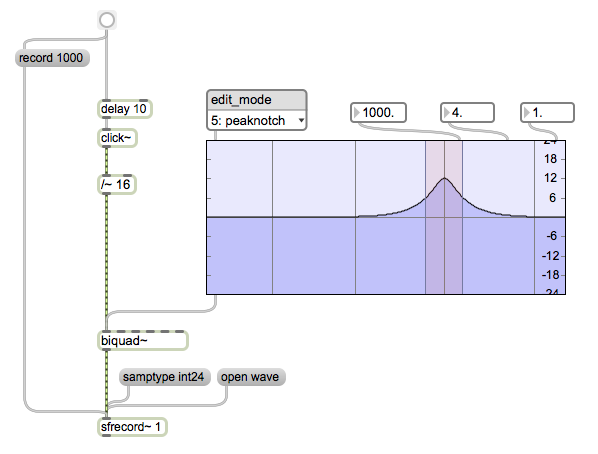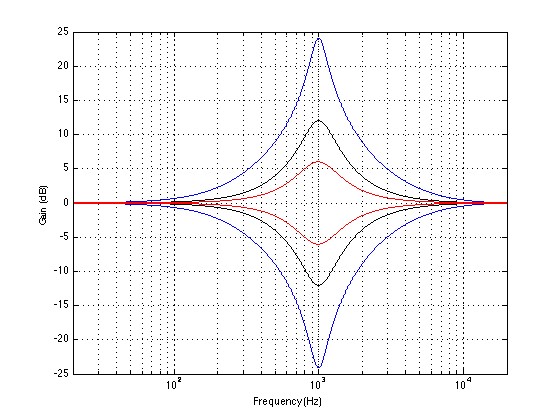# Q vs Q

I recently was interviewed by cycling74.com to talk about how I use Max/MSP when I’m doing the sound design for loudspeakers at Bang & Olufsen. I used part of the interview to “complain” gently about the fact that the filtergraph~ object in Max uses Robert Bristow-Johnson’s definition of “Q” without being explicit in stating that it does. (Don’t get me wrong here – I have no issues with RB-J’s definition – I just want to know what I’m using without having to find out for myself…)

Following the interview’s publication on the website, there was a discussion that ensued about this issue. So, I decided to put my money where my mouth is and to find out for sure what the filtergraph~ object is doing.

I could have done this by analysing the 5 coefficients that come out of the object. I decided not to do this – but to actually run an impulse through a biquad~ object to look at the whole filtergraph~ + biquad~ system. So, the patcher I put together to do the analysis looked like thisAdmittedly, it’s a bit quick-and-dirty, but it does the job. If you’re wondering, the only reason the /~ 16 object is there is to make sure I don’t clip the sfrecord~ object.

I kept the centre frequency (fc) at 1 kHz and then did 24 combinations of 6 gains (0.0625, 0.25, 0.5, 2, 4, and 16) and 4 Q’s (1, 2, 4, 16), each producing a single impulse response that I imported into Matlab for analysis.

So, the first question to answer is a rather simple one: Is the filtergraph~ object producing reciprocal peak/dip or constant Q filters when you set its type to “peaknotch”? We can quickly figure this out by putting two filters in series with reciprocal gains and identical centre frequencies and Q’s and looking at the output. Instead, I’ll just put up a plot.This plot shows 6 filter settings, all with fc=1 kHz and Q = 1. The linear gains are 0.0625, 0.25, 0.5, 2, 4, and 16. As you can see there, the filters with gains of 0.0625 and 16 (or -24 and +24 dB) are symmetrical. Therefore, we can reasonably conclude that we’re using reciprocal peak/dip filters and not constant Q.

Now to the meaty stuff. The question then is “exactly how is Q defined?” If you stay away from definitions of Q that involve damping or the S plane (see here), and we stick with the definitions that people who make filters use (i.e. see here as an example), then there are (kind of) seven different, but typical definitions of Q for a peaking filter. (Yes – SEVEN!) This is not because there are seven different equations for calculating Q. Q is calculated by dividing the frequency of the maximum of the peak in the magnitude response over the bandwidth of the boosted frequency band of the signal. (more simply expressed as Q = fc / bw). The problem is that the bandwidth is defined differently by different people, and therefore has different implementation in different gear.

If the frequency band being affected is a boost, then you have three possible definitions of bandwidth:

1. The bandwidth is the difference in Hz between the two frequencies that are 1/sqrt(2) lower (in other words, -3.01 dB or half of the power) in magnitude than the highest peak in the magnitude response.
2. But what do you do when the maximum peak is less than 3 db greater than the minimum magnitude? How do you find a -3 dB point? Well, in [Moorer, J. A. (1983). The manifold joys of conformal mapping: Applications to digital filtering in the studio. Journal of the Audio Engineering Society, 31(11):826–841.], Andy Moorer suggested that, in this case, we use a different definition where we look for the frequencies where the magnitude is one-half the value of the maximum magnitude (in dB). So, if the peak in the magnitude response is +4 dB and the minimum is 0 dB, we look for the +2 dB points. The difference between these two points is the bandwidth.
3. Robert Bristow-Johnson in his Cookbook and in [Bristow-Johnson, R. (1994). The equivalence of various methods of computing biquad coefficients for audio parametric equalizers. In 97th International Convention of the Audio Engineering Society. Audio Engineering Society.] rightly complains that this IF > 6.02 dB ELSE statement is confusing. So, he suggested that we just use the half-gain definition all of the time. So, if you have a boost of 12 dB, you look for the 6 dB points to get the bandwidth.

If the frequency band being affected is a cut, then you have four possible definitions.

1. The bandwidth is the difference in Hz between the two frequencies that are 1/sqrt(2) lower (in other words, -3.01 dB or half of the power) in magnitude than the highest peak in the magnitude response. This is interesting, because we’re looking at a cut – so the points we’re looking for are always -3.01 dB, regardless of the depth of the notch. This is what is known as a “constant Q” filter.
2. The bandwidth is the difference in Hz between the two frequencies that are 1/sqrt(2) higher (in other words, +3.01 dB or twice the power) in magnitude than the lowest dip in the magnitude response.
3. We use Andy Moorer’s IF / THEN statement, but we’re looking for the +3.01 dB points above the dip when it is lower than -6.02 dB.
4. We use Robert Bristow-Johnson’s definition and look for the mid-point between the maximum and minimum values in the magnitude response.

So, we have 3 options if the band is boosted, and 4 options if it’s a cut.

Enough of the back-story. Let’s look at how the filtergraph~ object behaves.

As I said, I set the fc to 1 kHz and did impulse response of the 24 combinations of 6 gains and 4 Q’s. I then converted the impulse responses to magnitude responses with 2^17-point FFT’s (giving me a frequency resolution of <1 Hz, since I did the impulse responses at 44.1 kHz). I then found out the Q of the results, using two definitions (the gain-midpoint, and the -3.01 dB definitions) of each. And the results are as follows:

 Linear Gain MSP Q Midpoint gain Q -3.01 dB Q 0.0625 1 1.0 1.0 0.25 1 1.0 0.5 0.5 1 1.0 0.3 2 1 1.0 1.0 4 1 1.0 1.9 16 1 1.0 4.0 0.0625 2 2.0 0.5 0.25 2 2.0 1.1 0.5 2 2.0 2.0 2 2 2.0 2.0 4 2 2.0 3.8 16 2 2.0 8.0 0.0625 4 4.0 1.0 0.25 4 4.0 2.1 0.5 4 4.0 4.0 2 4 4.0 4.0 4 4 4.0 7.5 16 4 4.0 16.1 0.0625 16 15.9 4.0 0.25 16 15.9 8.5 0.5 16 15.9 15.9 2 16 16.2 16.2 4 16 16.2 30.3 16 16 16.2 66.0

There are a few things to mention here:

• The “midpoint gain Q” values are slightly different at high “MSP Q” values, probably due to small errors caused by my limited resolution in the frequency domain. Please feel to ignore the fact that they are not identical to the “MSP Q”. Call it a measurement error.
• In the case of the gains of 2 and 0.5, the two Q’s will always match, since the -3.01 dB point is the gain-midpoint. (This is just a nice rule of thumb to remember – like the fact that -40° C = -40° F.)
• As you can see, the higher the Q and the higher the gain (or attenuation), the more the two definitions of Q diverge. This can be a problem if (taking me as an example) you are working with someone who does a measurement of a system that needs correction with a filter, and then you implement the correction with a filter. The only problem here is that they’re using the -3 dB definition and you’re using Max/MSP, for example… Bad things will happen if they want a Q of 16 but you’re giving them a Q of 66 (see the last line of the table above) – just because you think you’re saying the same thing but you’re not.
• Obviously (ignoring measurement error – see above) Max/MSP’s filtergraph~ object uses Robert Bristow-Johnson’s equations for the peaknotch type of filter.

N.B. If you need to convert from one system to another, I’ve described how to do this in this posting.

1. ##### christopher deckardsays:

Very informative — thanks for posting, Geoff! BTW, I’m currently in the early stages with some work whereby we’re able to update changes from MAX/MSP directly into the DSP inside the speaker. Feel free to drop me a line if you’re interested.

Cheers!

-Chris Deckard
Seattle, Wa

2. ##### geoffsays:

Hi Chris,
This is interesting – although you’re a bit vague. Are you controlling parameters in a pre-made DSP layout, or is the DSP layout itself coming from MSP? What kind of processor are you using in the loudspeaker?
Feel free to email directly if you don’t want too much info here…
cheers
-geoff

3. ##### r b-jsays:

hey Geoff, i’m back to “stalking” you. :-)

i didn’t realize until now that you had replied to my comment at the cycling74 site. that’s how i found my way here. anyway, you might want to review this:

“But what do you do when the maximum peak is less than 6.02 db greater than the minimum magnitude? How do you find a -3 dB point?”

actually, if the peak gain was 4 dB, the -3 dB points would be at +1 dB from the flat part. so there isn’t that much of a problem for defining 3-dB BW of sorts for a parametric EQ until the peak gain gets down to 3 dB.

the reason why Andy’s “IF-ELSE” definition switches at a peak (or valley) gain of 6.02 dB is because at that peak gain, both definitions; the -3 dB bandwidth and the half-dB bandwidth, agree.

i think the rhetorical question might better asked as: “But what do you do when the maximum peak is less than 3 dB greater than the minimum magnitude? How do you find a -3 dB point?”

i dropped into an AES session (i think a workshop) where they were discussing the meaning of Q or BW and such and i think that it might be good if *someone* wrote a standard guideline so that all the equipment or plug-ins would agree.

best regrads, Geoff. maybe i might run into you at the AES in NYC in a couple weeks?

L8r,

r b-j

4. ##### geoffsays:

Hi Robert,

Good point on the “less than 6.02 dB” line. I have changed the text accordingly to fix it.

I didn’t attend the convention in NY. However, if you ever do a workshop on Q and bandwidth, please let me know. I’d love to attend!

Cheers
-geoff

5. ##### Jason Coreysays:

Hey Geoff,

Cool stuff – thanks for posting. I was inspired by your post to do some measurements of other EQs and have put the info on my website: